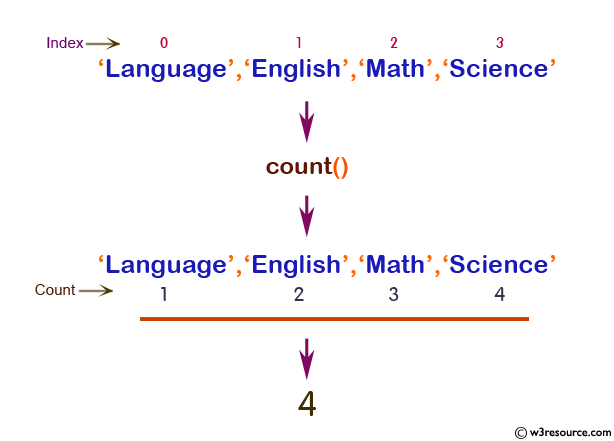# PHP: count() function

## PHP: Count all elements in an array

The count() function is used to count the elements of an array or the properties of an object.

Note: For objects, if you have SPL installed, you can hook into count() by implementing interface Countable. The interface has exactly one method, Countable::count(), which returns the return value for the count() function.

Version:

(PHP 4 and above)

Syntax:

`count(array_name, mode) `

Parameters:

Name Description Required /
Optional
Type
array_name Specifies the array or object to count. Required Array
mode Sets the mode of the function.
Possible values :
COUNT_RECURSIVE (or 1) : here the count() function counts the array recursively. This is useful for counting all the elements of a multidimensional array.
The default value is 0.
Optional Integer

Return value:

The number of elements in array_name.

Value Type: Array.

Note: The count() function may return 0 for a variable which is not set, but it may also return 0 for a variable that has been initialized with an empty array.
The isset() function should be used to test whether a variable is set or not.

Example :

``````<?php
\$a = 'Language';
\$a = 'English';
\$a = 'Math';
\$a = 'Science';
\$result = count(\$a);
echo \$result;
?>
``````

Output:

`4`

Pictorial Presentation:View the example in the browser

Practice here online :

PHP Function Reference

Previous: compact
Next: current

﻿

## PHP: Tips of the Day

PHP: How to convert string to boolean?

Strings always evaluate to boolean true unless they have a value that's considered "empty" by PHP (taken from the documentation for empty):

1. "" (an empty string);
2. "0" (0 as a string)

If you need to set a boolean based on the text value of a string, then you'll need to check for the presence or otherwise of that value.

`\$test_mode_mail = \$string === 'true'? true: false;`

EDIT: the above code is intended for clarity of understanding. In actual use the following code may be more appropriate:

`\$test_mode_mail = (\$string === 'true');`

or maybe use of the filter_var function may cover more boolean values:

`filter_var(\$string, FILTER_VALIDATE_BOOLEAN);`

filter_var covers a whole range of values, including the truthy values "true", "1", "yes" and "on".

Ref : https://bit.ly/33SQ3eP